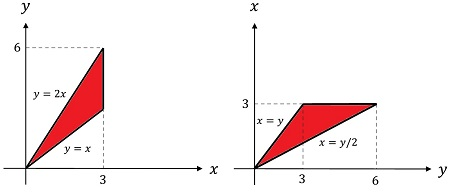# Let f(x, y) = x^2 e^{x^2} and let R be the triangle bounded by the lines x = 3, x = y/2, and y =...

## Question:

Let {eq}f(x, y) = x^2 e^{\large x^2} {/eq} and let R be the triangle bounded by the lines x = 3, x = y/2, and y = x in the xy-plane.

a. Express {eq}\int_R fdA {/eq} as a double integral in two different ways.

b. Evaluate one of them.

## Multiple integrals

Multiple integrals are very common in physics. The mass, the moment of inertia, the charge, etc. are computed using double or triple integral involving some density function depending on the coordinates. For example, the charge of a body is written as,

{eq}M=\displaystyle \iiint_R \rho(x,y,z) dxdydz {/eq},

the triple integral is calculated over the volume {eq}V {/eq} of the body and {eq}\rho(x,y,z) {/eq} is the charge density.

For superficial densities, the problem reduces to the computation of a double integral over the region {eq}R {/eq} covered by the body.

The diagram below shows the integration region. The first graph has the {eq}y {/eq} as dependent variable while the second has {eq}x {/eq} as the dependent variable. This distinction will be useful when performing the integration for either variable first.The variable {eq}x {/eq} covers the interval {eq}x\in\{0,3\} {/eq}, while the variable {eq}y\in\{0,6\} {/eq}.

a) The form of the double integral {eq}\int_R f dA {/eq} depends on the way we define the region, either in terms of {eq}x {/eq} or {eq}y {/eq}.

In general, the double integral takes the form,

{eq}I=\iint_{R} f(x,y) dx dy=\displaystyle\iint_{R} x^2e^{x^2} dx dy {/eq}.

Let us use the diagrams of the regions to obtain the integration limits of the double integrals.

x first integral

{eq}I_x=\displaystyle\int_{y\;limits} \left(\displaystyle\int_{x\;limits} x^2e^{x^2} dx\right) dy {/eq}.

In this case the {eq}x {/eq} limits are given by the lines {eq}x=y/2 {/eq} (lower limit), {eq}x=y {/eq} and {eq}x=3 {/eq} (upper limit). Notice that since the upper limit is not given by a single expression but by a sectionally defined function we must divide the integral into two parts,

{eq}I_x=\displaystyle\int_0^3 \left(\displaystyle\int_{y/2}^y x^2e^{x^2} dx\right) dy+\displaystyle\int_3^6 \left(\displaystyle\int_{y/2}^3 x^2e^{x^2} dx\right) dy \qquad\qquad (1) {/eq}.

The {eq}y {/eq} limits are {eq}0 {/eq} and {eq}6 {/eq}.

y first integral

Proceeding in the same way for the {eq}y {/eq} first integral,

{eq}I_y=\displaystyle\int_{x_limits} x^2e^{x^2} \left(\displaystyle\int_{y_limits} dy\right) dx {/eq}.

This time the {eq}y {/eq} limits are given by the lines {eq}y=x {/eq} (lower limit) and {eq}y=2x {/eq} (upper limit). The resulting integral takes the form,

Equations (1) and (2) are the forms of writing the double integral.

b) The integral computed in either order must give the same result.

{eq}I_x=I_y {/eq}.

Yet is way easier to use expression (2) and start by integrating the {eq}y {/eq} variable.

{eq}\begin{align} I_y&=\displaystyle\int_{0}^3 x^2e^{x^2} (\displaystyle\int_{x}^{2x} dy) dx \\ &=\displaystyle\int_{0}^3 x^2e^{x^2} (2x-x)dx\\ &=\displaystyle\int_{0}^3 x^3e^{x^2} dx \end{align} {/eq}.

After carrying out the {eq}y {/eq} integration the exponent of the {eq}x {/eq} terms raises to three. Arranging the integral,

{eq}I_y=\displaystyle\int_{0}^3 \dfrac{x^2e^{x^2}}{2} (2xdx) {/eq},

the term inside the parenthesis is the differential of {eq}x^2 {/eq},

{eq}I_y=\displaystyle\int_{0}^3 \dfrac{x^2e^{x^2}}{2} (dx^2) {/eq}.

Changing variables,

{eq}u=x^2 {/eq},

{eq}I_y=\displaystyle\int_{0}^3 \dfrac{x^2e^{x^2}}{2} (dx^2) {/eq}.

Changing varibles with {eq}u=x^2 {/eq}. The new integration limits are {eq}0 {/eq} and {eq}9 {/eq},

{eq}I_y=\displaystyle\int_0^9 \dfrac{ue^{u}}{2} u {/eq},

integrating by parts with {eq}f=u {/eq} and {eq}dg=e^u {/eq},

{eq}\begin{align} I_y&=fg-\int gdf\\ &=\dfrac{1}{2}ue^u\bigg|_0^9-\dfrac{1}{2}\int_0^9 e^u\\ &=\dfrac{9}{2}e^9-\dfrac{1}{2}e^9\\ &=\boxed{4e^9} \end{align} {/eq}.